# Homomorphism

(Redirected from Strong homomorphism)

A morphism in a category of algebraic systems (cf. Algebraic system). It is a mapping of an algebraic systemthat preserves the basic operations and the basic relations. More exactly, let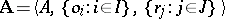be an algebraic system with basic operations,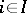, and with basic relations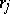,. A homomorphism from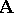into a system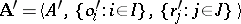of the same type is a mapping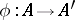that satisfies the following two conditions:(1)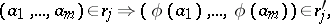(2)

for all elements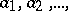fromand all,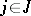.

E.g., ifis a group andis a normal subgroup of it, then by assigning to each element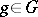its cosetone obtains a homomorphismfromonto the quotient group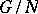.

Suppose that each elementfromis brought into correspondence with some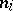-ary function symbol, while each elementfrom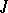is brought into correspondence with an-place predicate symbol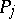, and suppose that in each system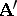of the same type asthe result of the-th basic operation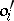, applied to the elementsfrom, is written as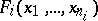, while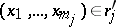is denoted by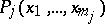. Conditions (1), (2) are then simplified and take the form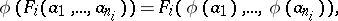A homomorphismis called strong if for any elements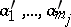from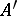and for any predicate symbol,, the condition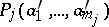implies that there exist elements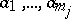insuch that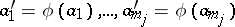, and such that the relationholds.

In the case of algebras the concepts of a homomorphism and a strong homomorphism coincide. For models there exist homomorphisms that are not strong, and one-to-one homomorphisms that are not isomorphisms (cf. Isomorphism).

Ifis a homomorphism of an algebraic systeminto an algebraic systemandis the kernel congruence of, then the mapping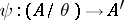defined by the formula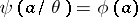is a homomorphism of the quotient systeminto. If, in addition,is a strong homomorphism, thenis an isomorphism. This is one of the most general formulations of the homomorphism theorem.

It should be noted that the name "homomorphism" is sometimes applied to morphisms in categories other than categories of algebraic systems (homomorphisms of graphs, sheaves, Lie groups).